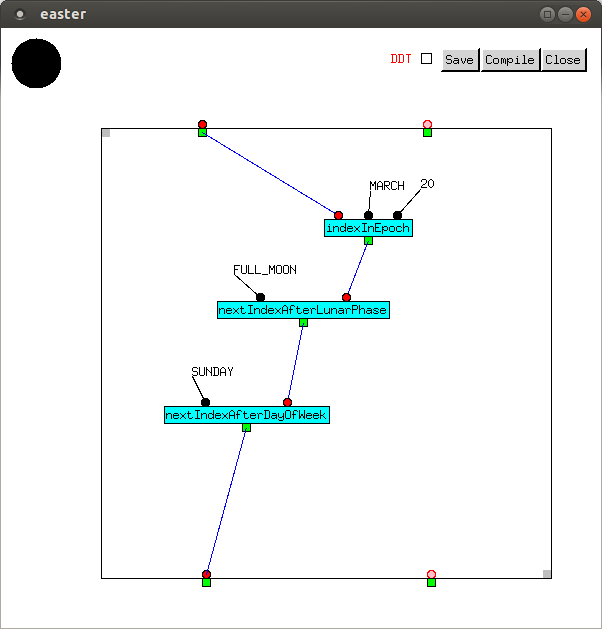# The Gregorian Calendar

## Introduction

Handling dates isn't trivial. Here, we implement an API for the Gregorian Calendar. This isn't helpful for calculating when Chinese New Year, Passover, or Ramadan falls, but for most purposes it will suffice. The sources of complexity in the Gregorian calendar are in determining whether a given year is leap, and the different month lengths.

To simplify matters, we begin the calendar at a date (the `EPOCH`) set by the user, for example Sunday, 1st January 1950. Dates before this aren't handled. The API contains three top-level functions: `indexInEpoch`, which converts a given date into the number of days from the epoch; `yearMonthDay`, which converts back to a date, and `dayOfWeek`, which displays the day of the week.

In the C library time.h, months start at 0 and days of the month start at 1. That is brain-damaged. Here, we will follow the ISO date convention. Months and days of the month both start at 1. Days of the week also start at 1 (Monday). Names of months and days of the week can be returned simply by array look-up.

## Data Types

First, we define `Year`, `Month`, and `Day` types. These are implemented as subtypes of `Int`. This is safer than using `Int`, but the extra type safety it gives us is limited. Full Metal Jacket doesn't yet support subranges of `Int`, but these will be added later.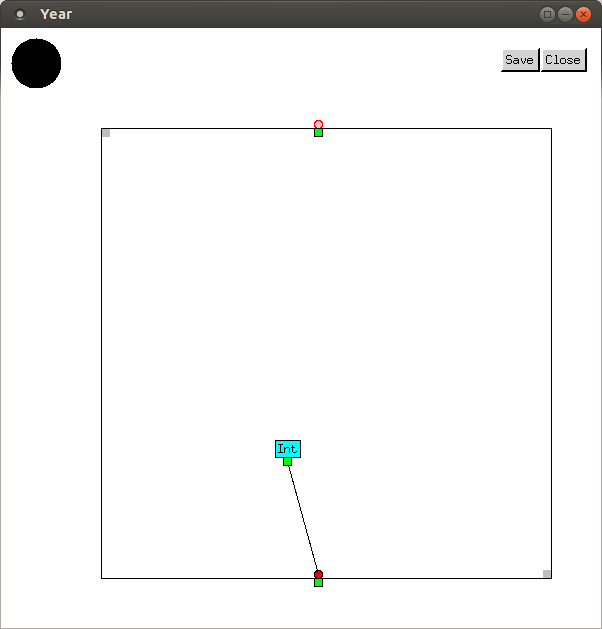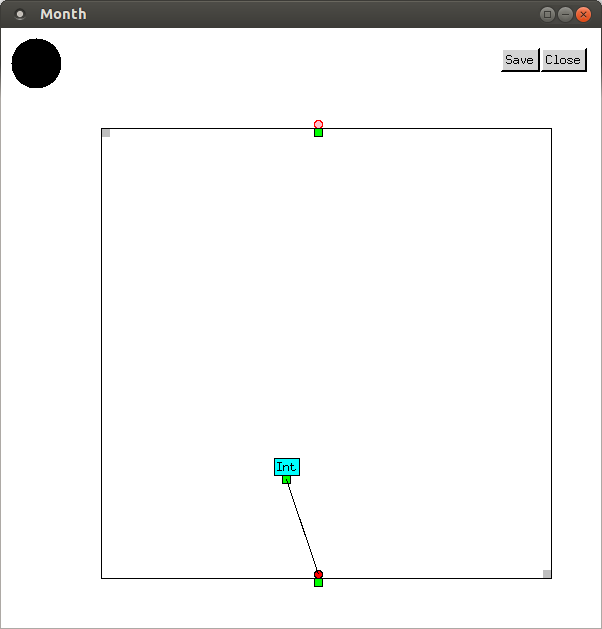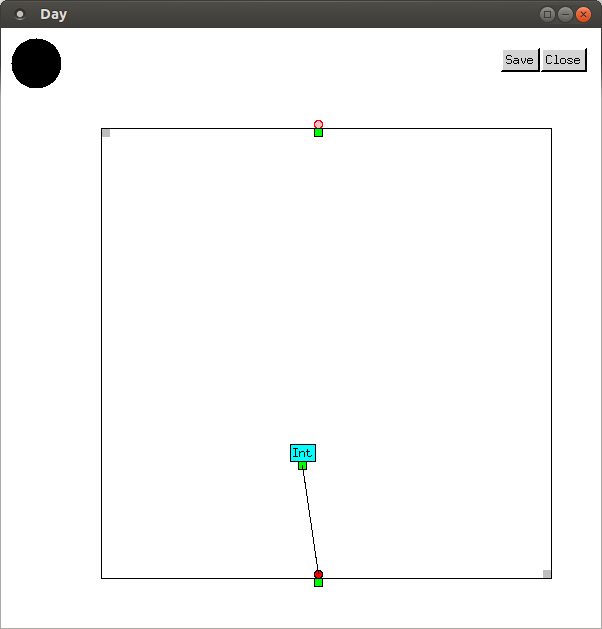## Functions

A leap year is divisible by 4, unless it's divisible by 100 but not 400. To make the code clearer, we first define a `divisibleByP` function, which outputs `T` if its first input, an `Int`, is exactly divisible by its second input, another `Int`, and otherwise outputs `NIL`.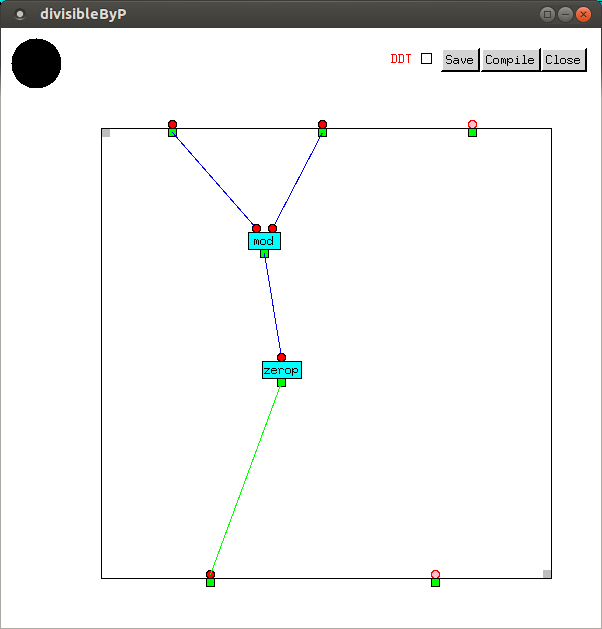We can now define `leapP`, which accepts a `Year` as its input, and outputs a `Bool`. `TRUE` and `FALSE` are names for `T` and `NIL` respectively, but with Boolean type.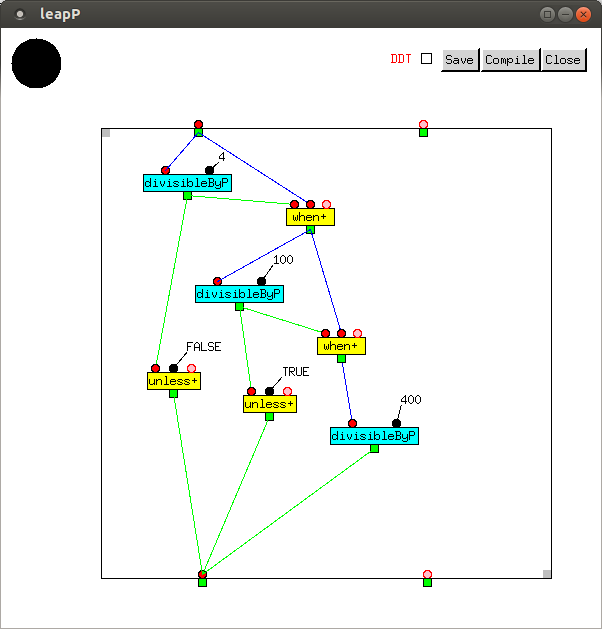The number of days depends on whether the year is leap.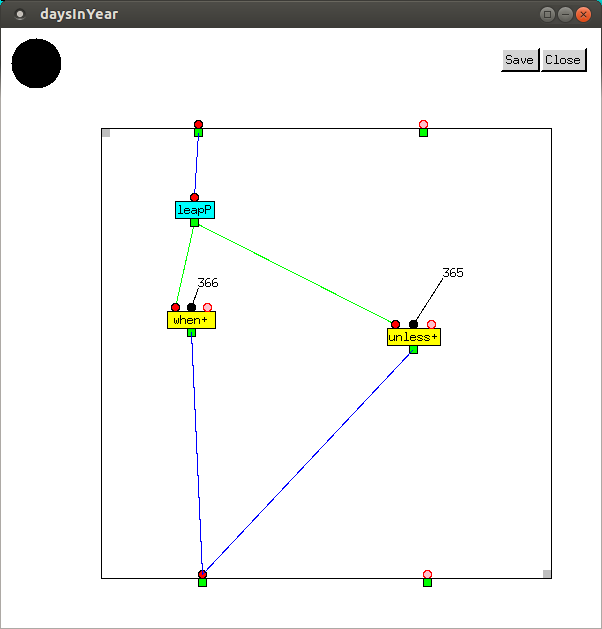The number of days before any month before March is the the same in any year. From March onwards, it's one more if the year is leap. The vector supplied to `elt` contains the number of days before any month if the year isn't leap.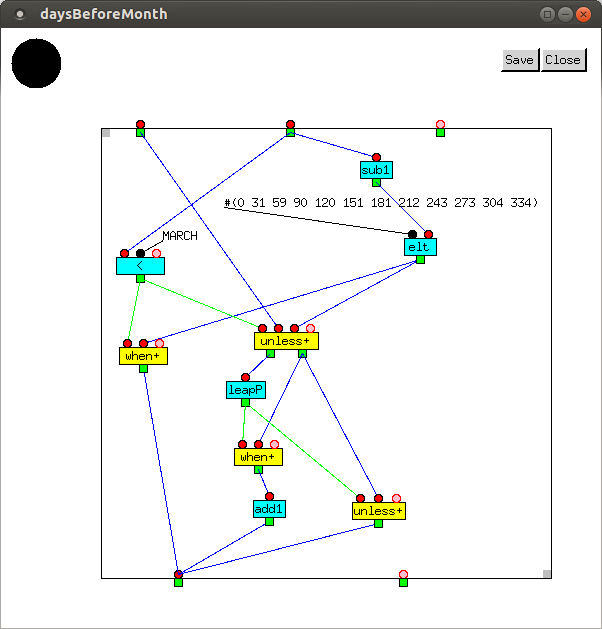`indexInYear` returns the index of a day in a given year, i.e. the number of days before it.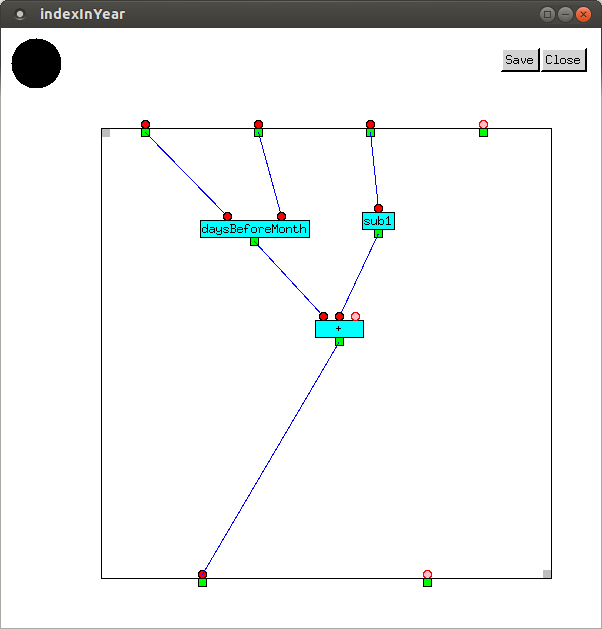The inputs of `indexInEpoch` are of type `Year`, `Month`, and `Day`. It outputs the number of days from the start of the epoch.

The first value in the loop is a year, initially set to `EPOCH` and incremented by one in each iteration. The second value is the date index, initially set to 0 (its value at `EPOCH`), and repeatedly incremented by the number of days in each year starting from `EPOCH`. When the year which was input has been reached, the value output by `indexInYear` is added to it.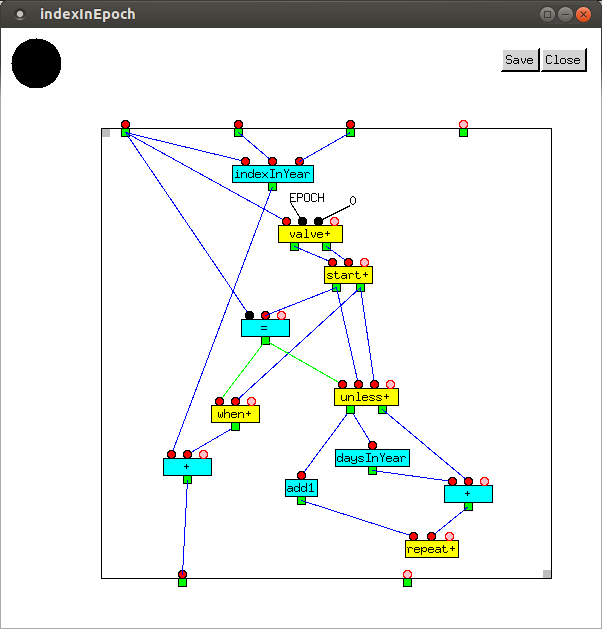`indexAndYearFromIndexInEpoch` takes the number of days since `EPOCH` as its input, and outputs the number of days since new year's day, and the year.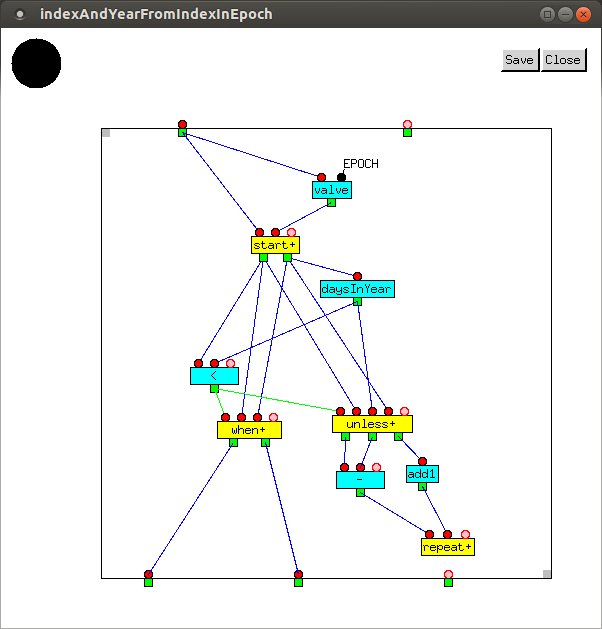`monthFromIndexInYear`takes the number of days since new year's day and the year as its inputs, and outputs the month. Each time around the loop, `daysBeforeMonth` is called, and the value it outputs is compared against the number of days since New Year.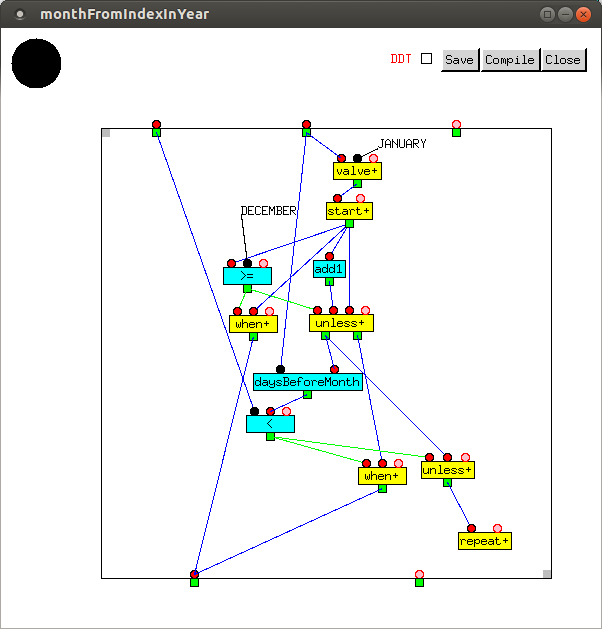`dayFromIndexInYear` takes the number of days since new year's day, the year, and the month, as its inputs, and outputs the day of the month.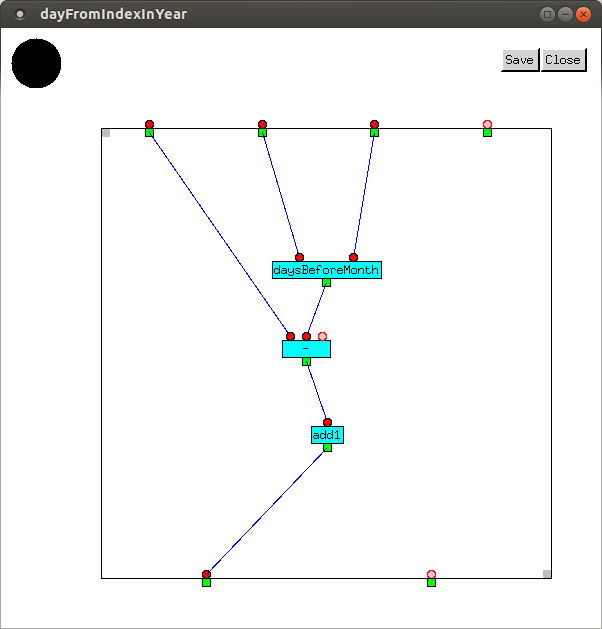`yearMonthDay` takes number of days since `EPOCH` as its input.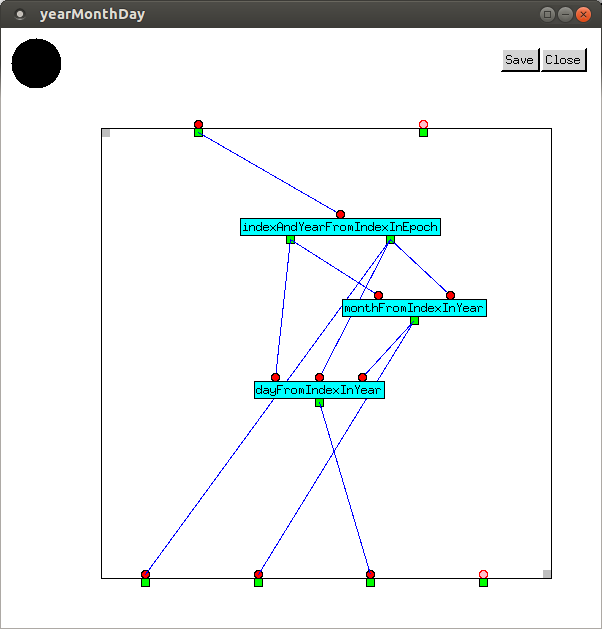The day of the week is just the remainder of the number of days since the start of `EPOCH`, except that it is 7 if the remainder is zero.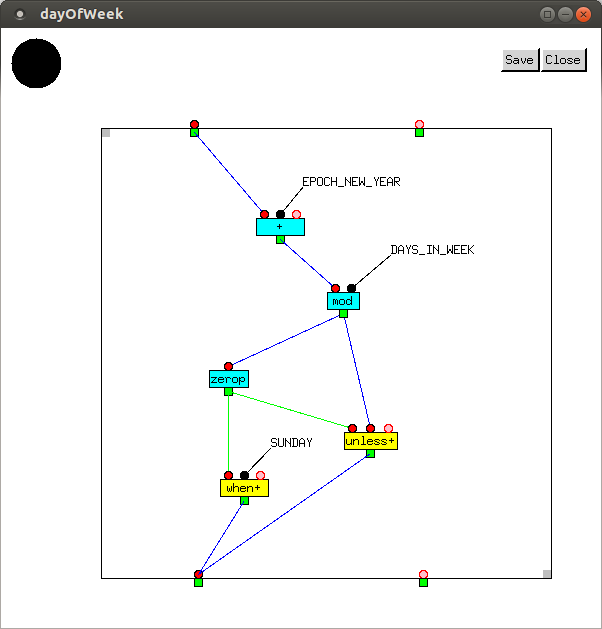In 2016, Easter falls on March 27th. It is, of course, a Sunday. The code in the sandbox outputs the number of days since `EPOCH`, then the year, month, and day of the month, followed by day of the week.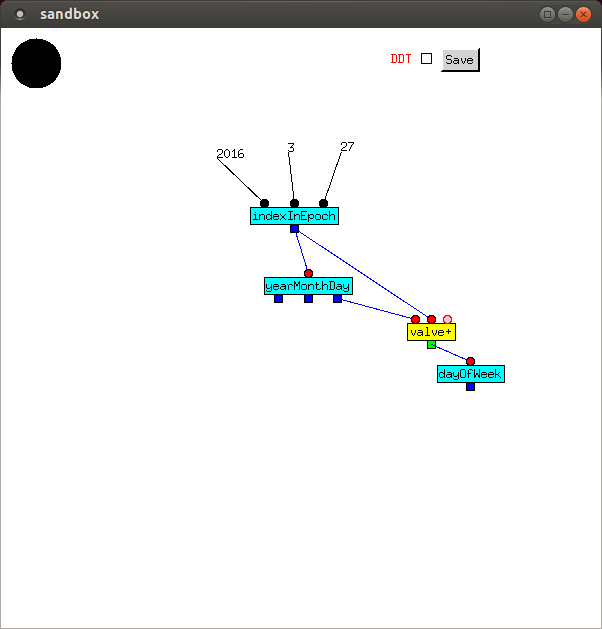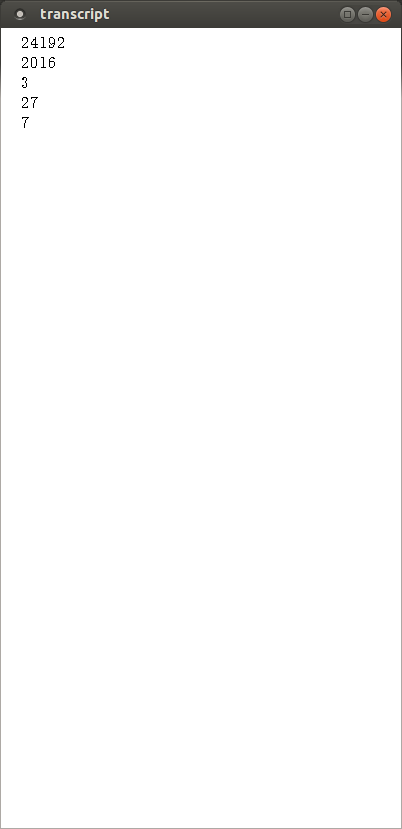## Bell, Book, and Candle

Determining the date on which Easter falls in a given year has always been problematic. At one point, an entire branch of the early Christian Church was threatened with excommunication over of a disagreement about how to calculate it. Computus, the algorithm currently used, is quite hairy and practically incomprehensible. Even Gauss got it wrong. There have been recent attempts to introduce a simpler mechanism.

In principle, the rule for calculating Easter is quite simple: Easter falls on the Sunday after the full moon after the vernal equinox, which close to `EPOCH` generally falls on March 20th. At the risk of being excommunicated, I will now present a simplified algorithm, which I have to say is wrong in 2008 and 2025. (For this, I make no apology: if ever there was a case of accidental difficulty, the currently accepted algorithm for Easter is it.)

First, we write a simple helper function to calculate the difference between the value and the closest integer not higher than it.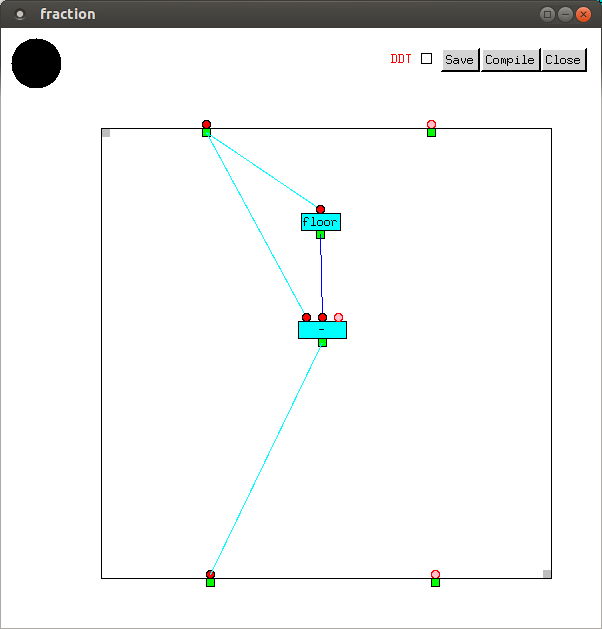The synodic month (i.e. the time between a given phase and the next occurrence of it) varies only slightly, and averages `29.530588` days. So if we know the lunar phase at the `EPOCH`, calculating it on any later date is straightforward. The value returned can be any `Real` between `0.0` up to but not including `1.0`, and is defined so that

 Phase Value New moon 0.0 First quarter 0.25 Full moon 0.5 Third quarter 0.75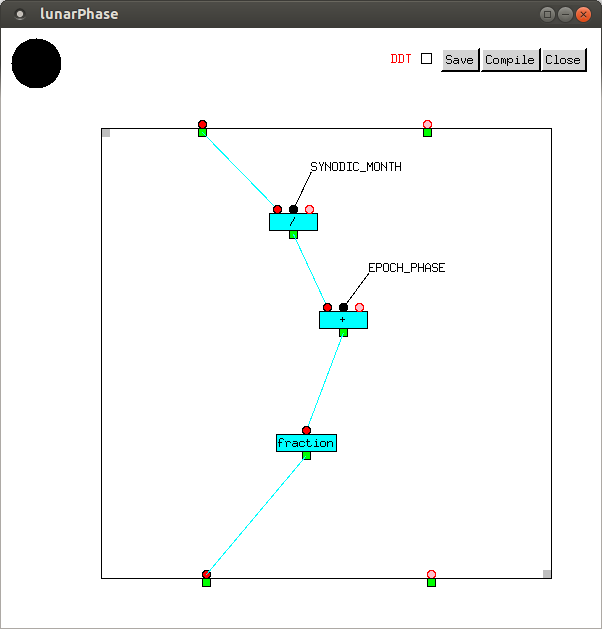Given a day of the week (the first argument) and an index (the second argument), the next index after the day of the week is obtained by iteration, starting one day after the second argument, until the day of the week is equal to the first argument.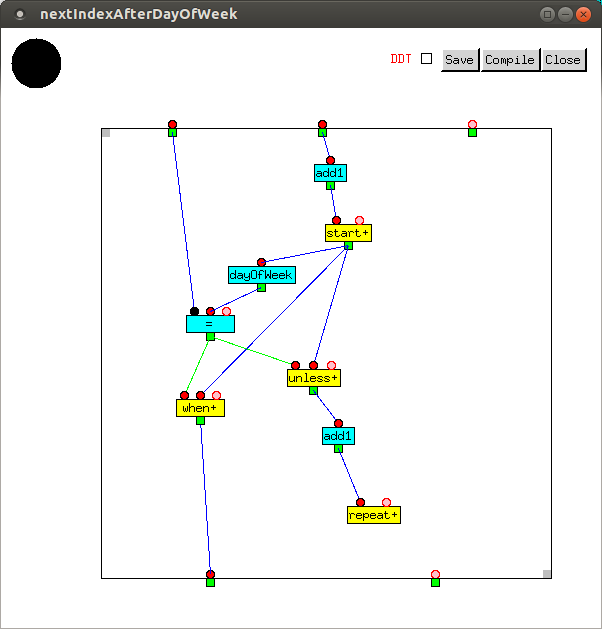The day after a given lunar phase is tricky because phases wrap around to `0.0` when a new moon is reached. This means we should iterate starting from the difference between the lunar phase on the day after the second argument, until `1.0` is reached or passed, and return the index when this happens. `DAILY_LUNAR_PHASE_CHANGE` is the inverse of `SYNODIC_MONTH`.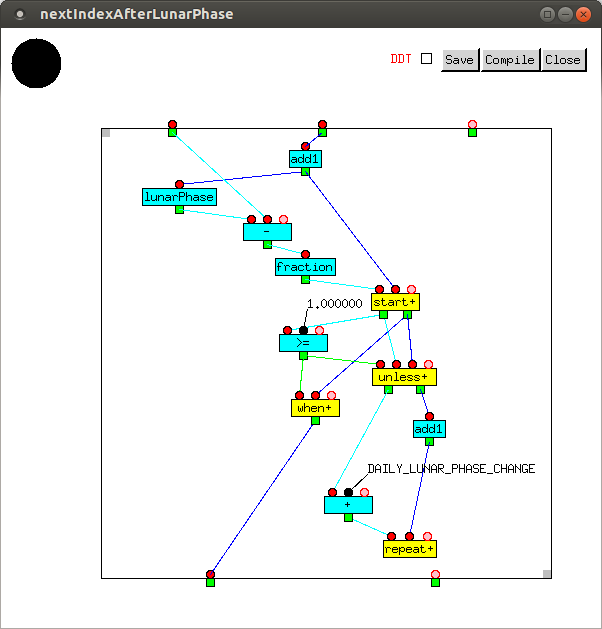`easter` returns the index of the Sunday after the full moon which follows the vernal equinox, as explained above. The constant `FULL_MOON` has value `0.5`.